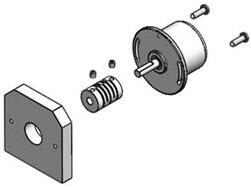# Angle Sensor Working Examples and Selection Points

### 1 Angle Sensor Rotating Principle

Angle sensors are used to detect angular movement and are composed of sensitive components, measuring circuits, smart components and interface components. Among them, detecting the value reflecting the angular position is the initial conversion unit of the sensor. There is a hole in the sensor that can match the axis of most devices when installation. When connected to the device, the angle sensor counts every setting revolution of the shaft based on sensor instructions. Because the number of counts is related to the initial position of the angle sensor. So when the angle sensor is initialized, its count value is usually set to 0. Of course, you can still reset the set value range.Angle Sensor Size

### 2 Angle Sensor Applications

An angle sensor is an electronic component that uses angle changes to locate the position of an object. It is suitable for the servo systems of automobiles, construction machinery, space devices, aircraft radar antennas, injection moulding machines, woodworking machinery, printing presses, electronic rulers, robots, engineering monitoring, computer-controlled sports equipment and other occasions which require precise displacement measurement.Angle Sensor Structure

### 3 Examples Analysis

The following is a specific applying example:

If the angle sensor is connected to any drive shaft between the motor and the wheel, the correct transmission ratio must be included in the read data. Here are some details by an example. On a robot, the motor is connected to the main wheels with a 3:1 gear ratio. The angle sensor is directly connected to the motor, so its transmission ratio to the driving wheel is also 3:1. In other words, the angle sensor counts three times, and the active wheel rotates once. The angle sensor counts 16 units per revolution, so 16*3=48 increments are equivalent to one revolution of the driving wheel. Now, we need to know the circumference of the gear to calculate the distance traveled. Pay attention, every LEGO gear tire will have its own diameter marked on it.

We chose a wheel with a shaft with a large volume, with a diameter of 81.6cm (Lego uses metric units), so its circumference is 81.6×π=81.6×3.14≈256.22cm. Now all the known quantities are available: the running distance of the gear is divided by 48 by the increment recorded by the angle and then multiplied by 256.

In the formula, R is the resolution of the angle sensor (counting value per revolution), and G is the transmission ratio between the angle sensor and the gear. We define it as the increment of the angle sensor for one rotation of the wheel. which is:

### I=G×R

In the example, G is 3, and for Lego angle sensors, R is always 16. Therefore, we can get:

### I=3×16=48

Each time it rotates, the distance the gear travels is exactly its circumference C. Applying this equation and using its diameter, you can get this conclusion.

### C=D×π

In our example: C=81.6×3.14=256.22

The last step is to convert the data recorded by the sensor -S into the distance of wheel movement -T, using the following equation:

### T=S×C/I

If the value read by the photoelectric sensor is 296, the corresponding distance can be calculated: T=296×256.22/48=1580, and the unit of distance (T) is the same as the unit of wheel diameter.Hall Angle Sensor Size

What’s more, using angle sensors to control your device wheels can find problems indirectly. For example, if the motor angle sensor operates normally, but the gear does not rotate, it indicates that your has problems with the sensor operation. This method is visual and time-saving.

#### Sensor Selection: 4 Elements

• High Sensitivity
• Fast Frequency Response
• Wide Linear Range
• High Stability

#### Angle Sensor Types

• Inclination Sensor
• Angular Velocity Sensor
• Angular Acceleration Sensor

### Angle Sensor Model Recommendation

The 0-10V series angle sensor adopts Hall effect sensor technology. According to Hall effect magnetic rule, the Hall signal is processed through a differential full analogue processing chain, using the classic drift voltage cancellation technology (Hall element four-phase rotation and chopper amplifier). It can reach 14Bit resolution (digital signal).

Its magnetic field intensity changes are caused by the magnetic field gap changes. In addition, its temperature changes and ageing factors will all act on the two signals equally, so the obtained angle signal has the characteristic of adaptive compensation. The accuracy of the temperature change of 0-10V series sensors is greatly improved.

### Conclusion

According to above mentioned, you should select a correct angle sensor. When you have high requirements for measuring output signal, you can choose a 4-20mA angle sensor. If the voltage is a key index, the current-type angle sensor is more reliable for the signal stability. In addition, the digital communication angle sensor is also divided according to different requirements. There are many types, commonly used are TTL (suitable for integrated development of single chip microcomputer), 232 (suitable for secondary development of upper computer), 485 (suitable for the development of control equipment). The rule is that different communication interface levels are used in different environments. The main determining factors are your own needs and applying cost.

I'm a electronic editor interested in semiconductor as my work. Hope to share and get new ideas from here, if you have any interset of my electronic works, you can visit https://www.kynix.com/Blog/.